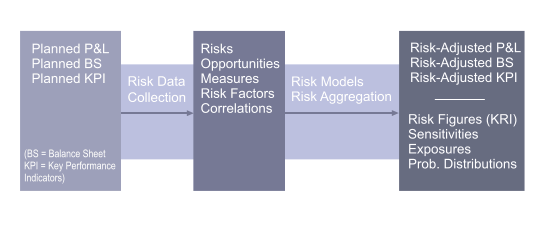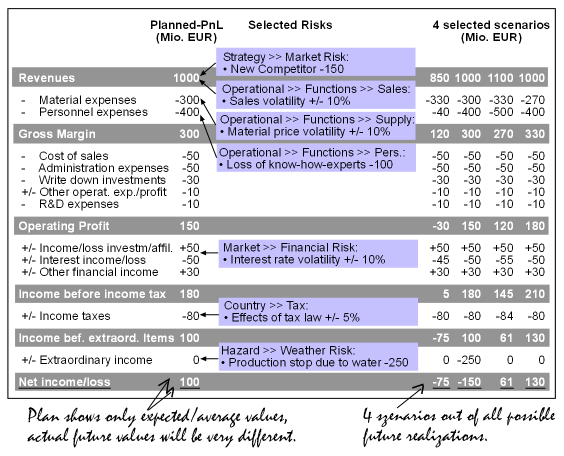﻿ N&N Risk ControllingRisk Controlling Goes Beyond Risk Documentation and Calculation of Risk AveragesContent: Risk Controlling Resolves the Following Problems

The risk controlling system from Noetzold & Noetzold goes far beyond risk documentation. Questions that will be answered by risk controlling are:

• How much risk is contained in planned return / cash flow / earnings?
• What are the top risks (and risk bundles) and what are the corresponding optimal steering / mitigation measures?
• Is the existing economic capital adequate for the current risk position or are resources wasted due to imbalance?
• Is the company threatened by hidden internal and / or external risks (e.g. single risks, risk bundles, risk driver)?
• Are liquidity, earnings, and assets correlated with volatile risk drivers (e.g. sensitivities)?
• What is the probability to generate the defined / planned minimum cash flow?
• Does the company or any corporate unit have risk bundle exposures (= exposures of correlated risks with likely simultaneous occurrence and extreme loss)?
• What are the risk diversification and concentration effects on different corporate consolidation levels?
• Are the risk exposures on each corporate level consistent and optimized with respect to the risk appetite of the whole company?

Objectives: Integrating the Risk Dimension into Planning Processes and KPIs

The objectives of risk controlling are:

• Identification and Quantification of risk exposures contained in plans.
• Reduction of volatilities in planned figures (e.g. reduction of earnings volatility).
• Identification of risks with extreme losses endangering the existence of the company.
• Optimal steering of the risks, risk bundles, and risk drivers.
• Generation of P&L positions of holding, corporate units, and business units under risk-return aspects.
• Calculation of unexpected losses / earnings and their probabilities.
• Definition of risk limits.
• Early warning for internal and external risks and risk drivers impact on key performance indicators.

Problems: Pitfalls in Risk Controlling

An extensive market study Corporate Risk Management and its Integration into Operational Business sponsored by Noetzold & Noetzold identified some common problems in risk controlling:

• Conventional addition of risks (result: overestimation of risks because no diversification effects are considered).
• Missing or incorrect risk aggregation (result: incorrect consolidation of risks; incorrect calculation of risk figures).
• Zero-One-Reduced Correlations (result: overestimation and/or underestimation of risks; unpredictable error in risk figures).
• Unrealistic distribution functions (result: erroneous risk figures, in particular, underestimation of extreme events, severe errors in predicting Value-at-Risk, Expected Loss, and quantiles)
• Incorrect time-dependence of risks (result: erroneous risk figures when calculating over extended periods, e.g. for projects; incorrect roll-over between time intervals)
• Use of standard Monte Carlo simulators (result: lack of precision/performance leading to severe errors in predicting Value-at-Risk, Expected Loss, and quantiles;
• Missing risk models (result: incorrect aggregation of risks of different type; severe errors in predicting Value-at-Risk, Expected Loss, and quantiles).
• Use of average values for risk management (result: risk average values are not risk figures, they only adjust planned values by expected or average values, they do not consider the full spectrum of all possible (not generally expected, not averaged) outcomes; risk mitigation and steering with risk average values is not risk controlling).
• Incomplete quantitative risk data (result: incorrect consolidation of risks; qualitative or incomplete quantitative risk data cannot be a basis for aggregation or simulation; risks have to be quantified, at least with losses and probabilities).
• Monitoring of individual risk data (result: unmonitored actual risk exposures; even for constant risk data bases, risk exposures can change due to evolving external risk drivers).

Solutions: Correct Risk Results Require Advanced Risk Models with Precise Risk Aggregation

The functionality and quality of risk controlling depends on four basic ingredients that are also the basis of the Opture® risk management software:

• Risk data collection methods (fully quantitative risk data base, experienced risk profilers, coherent scenario based profiling).
• Advanced risk management models (different risk types to represent risk events and market fluctuations, including interdependencies, consistent mathematical models).
• Correct risk aggregation (requires high-performance high-precision Monte Carlo simulator).
• Efficient software solution (user friendly intuitive interface, compatible with corporate environment).

Advanced risk management models with risk aggregation (based on simulation) are necessary to obtain correct consolidated risk results. The calculation of risk average values (= loss * probability) alone cannot be a basis of professional risk controlling or management. A risk average value merely adjusts the planned average value and does not say anything about volatilities or probabilities, i.e. it does not consider the full spectrum of possible scenarios that determine Value-at-Risk, Expected Loss, etc. Even imprecise risk aggregation can easily result in large (i.e. orders of magnitude) errors for risk figures that relate to extreme events, such as Value-at-Risk and Expected Loss.

Results: Basis for Risk-Adjusted Planning and Optimal Risk Mitigation

The risk controlling generates the basic data for an optimal risk mitigation and constitutes the basis for risk management. The results of risk controlling are:

• Risk-adjusted P&L statement and balance sheet for holding and corporate units.
• Risk figures (e.g. sigma, VaR, CFaR, EaR, RaC, RAROC, RORAC, Expected Loss, quantiles, probability distributions).
• Diversification effects, concentrations effects, and risk bundles (= correlated risks with simultaneous occurrence and extreme losses).
• Risk and risk driver concentrations, contributions, and sensitivities.
• Risk ranking and risk map.
• Optimal risk mitigation measures for individual risks and on corporate level.
• Thresholds for the limit system.
• Monitoring time evolution of different risk figures for individual risks, risk portfolios, risk driver, risk-adjusted P&L positions, etc.